# Introduction¶

This notebook shows basic utilities to load and process the greece_wildfire_datacube with xarray.

It contains basic instructions showing how to access, process and visualize the data.

First of all, we download the datacube, which resides in Zenodo in the form of a single netcdf file named dataset_greece.nc

In [ ]:
!wget -O dataset_greece.nc https://zenodo.org/record/4943354/files/dataset_greece.nc?download=1

--2021-06-29 12:20:00--  https://zenodo.org/record/4943354/files/dataset_greece.nc?download=1
Resolving zenodo.org (zenodo.org)... 137.138.76.77
Connecting to zenodo.org (zenodo.org)|137.138.76.77|:443... connected.
HTTP request sent, awaiting response... 200 OK
Length: 10487991499 (9.8G) [application/octet-stream]

dataset_greece.nc?d 100%[===================>]   9.77G  10.7MB/s    in 19m 39s



# Initial Imports¶

We start by importing the libraries we need. Make sure to have them installed in your system.

In [ ]:
import xarray as xr
import numpy as np
import matplotlib.pyplot as plt


# Access Data¶

Let's open the dataset with xarray

In [ ]:
ds = xr.open_dataset('./dataset_greece.nc')


We see that the dataset has x,y and time dimensions.

More specifically it contains 4314 days (from 06/03/2009 to 06/12/2020) of 700x562 rasters

Dynamic variables like the burned_areas have all three dimensions, while static variables like clc_2012 misses the temporal component and only has x, y dimensions.

# Basic Plots¶

In this section, we'll see some basic functionality showing how to select and visualize the variables in the datacube.

## Corine Land Cover¶

Corine Land Cover 2012 (clc_2012) is a static dataset, containing Land Cover values . Let's see what it looks like

In [ ]:
ds['clc_2012'].plot(cmap='Spectral', vmin=0, vmax=44, figsize=(10,8))

Out[ ]:
<matplotlib.collections.QuadMesh at 0x7f0273a64190>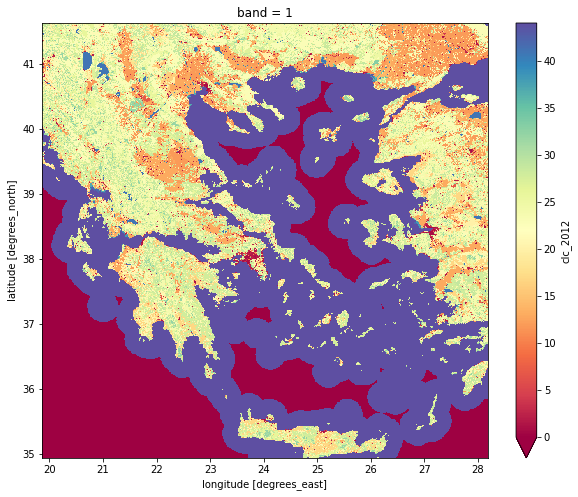Maximum Temperature for a specific period of time for a specific pixel (300x300) in the dataset. We can plot both the time-series of the temperature and a histogram of the values

In [ ]:
fig, axes = plt.subplots(ncols=2, figsize=(10,4))
(ds['era5_max_t2m']).isel(x=300, y=300).plot(ax=axes)
(ds['era5_max_t2m']).isel(x=300, y=300).plot.hist(ax=axes)
plt.tight_layout()
plt.draw()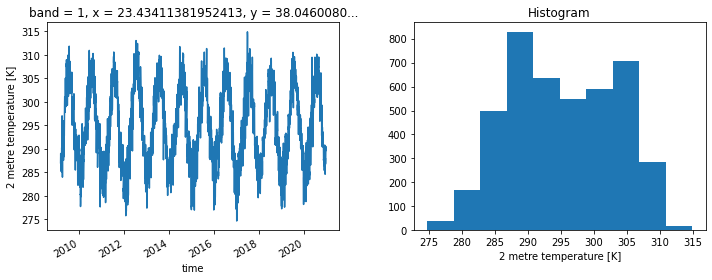# The same but only for year 2019¶

In [ ]:
fig, axes = plt.subplots(ncols=2, figsize=(10,4))
ds['era5_max_t2m'].sel(time=slice("2019-01-01", "2019-12-31")).isel(x=300, y=300).plot(ax=axes)
ds['era5_max_t2m'].sel(time=slice("2019-01-01", "2019-12-31")).isel(x=300, y=300).plot.hist(ax=axes)
plt.show()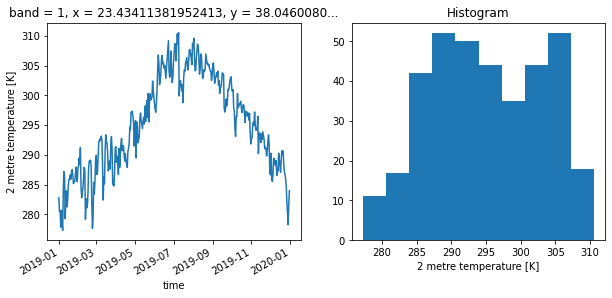A 10-rolling mean of the NDVI for a specific period of time and a specific pixel

In [ ]:
ds['1 km 16 days NDVI'].isel(dict(time=slice(4000, 4200), x=300, y=300)).rolling(time=10, center=True).mean().plot()

Out[ ]:
[<matplotlib.lines.Line2D at 0x7f0273749210>]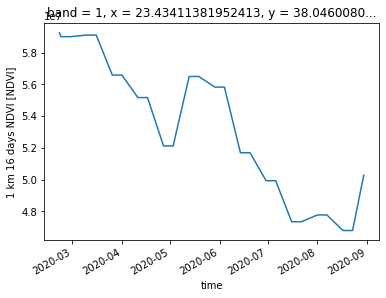Plots of land surface temperature for a series of days for the whole Greece region

In [ ]:
ds['LST_Day_1km'].isel(time=slice(4157, 4166)).plot(x="x", y="y", col="time", col_wrap=3)

Out[ ]:
<xarray.plot.facetgrid.FacetGrid at 0x7f02736b3790>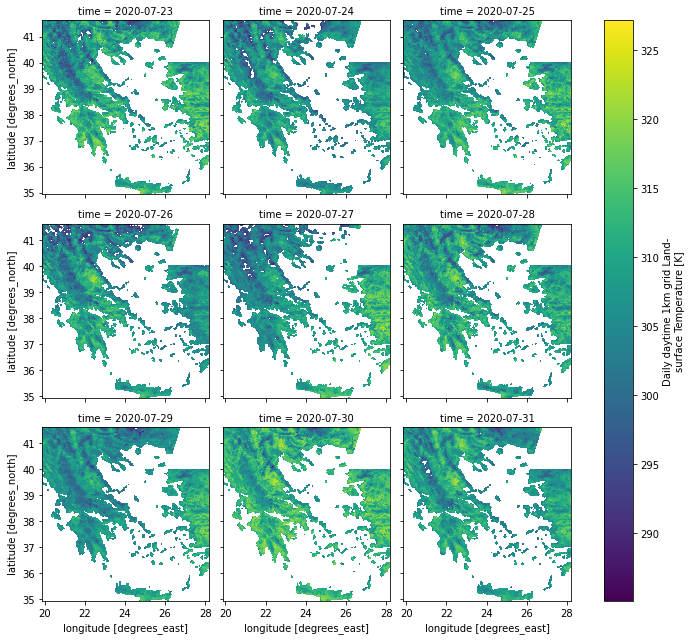We can plot the fire weather index for the same days

In [ ]:
ds['fwi'].isel(time=slice(4157, 4166)).plot(x="x", y="y", col="time", col_wrap=3)

Out[ ]:
<xarray.plot.facetgrid.FacetGrid at 0x7f93a215f3d0>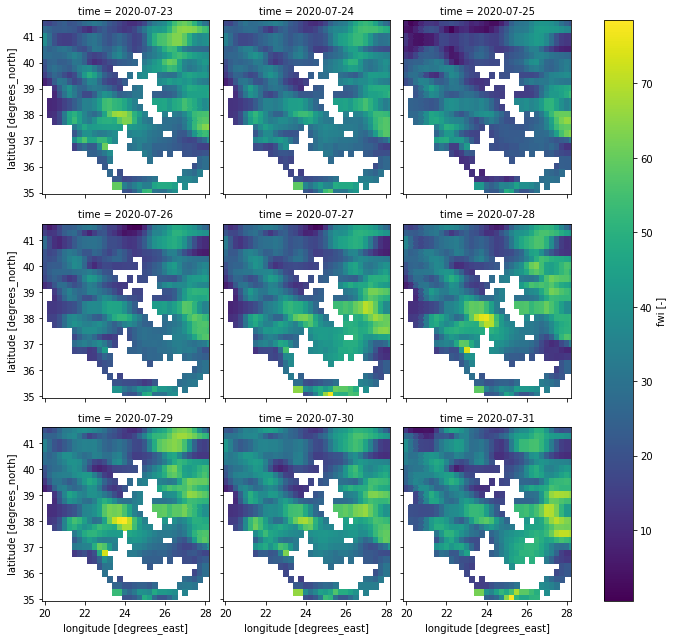Or the burned areas from a fire that started those days

In [ ]:
ds['burned_areas'].isel(time=slice(4157, 4166)).plot(x="x", y="y", col="time", col_wrap=3)

Out[ ]:
<xarray.plot.facetgrid.FacetGrid at 0x7f938942d550>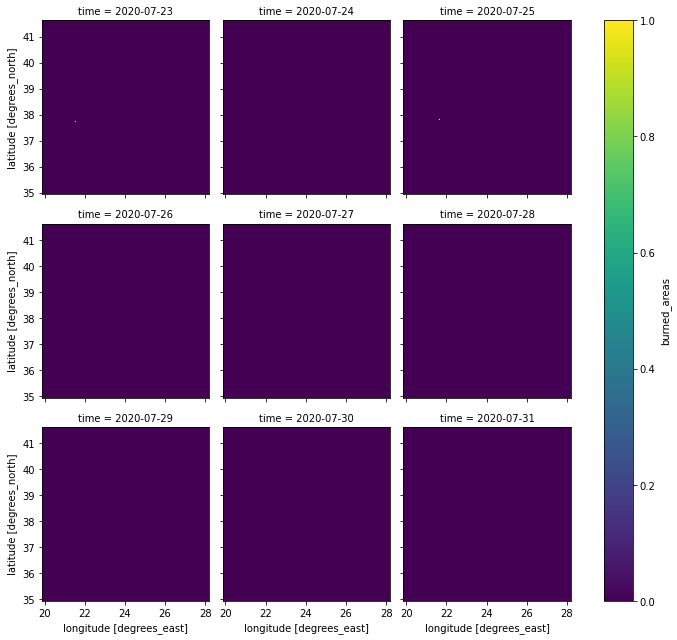Just a few pixels of burned areas. It is going to be quite challenging to model that... Stay tuned for our first models in the next notebooks that we will publish.

# Discussion¶

We saw how easy it is to access, process and visualize data in the datacube, even if the actual data is very large and cannot be loaded in memory.

In a later notebook we'll see how to develop some ML models using our datacube to predict the burnt area from next day's fire. Stay tuned!

Notebook Authors: Ioannis Prapas (https://github.com/iprapas), Spyros Kondylatos (https://github.com/skondylatos)